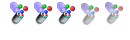## MCAT practice questions

MCAT preparation and implications of MCAT scores.

Moderators: cheechootrain, CaribMD, AL_emt

### Re: MCAT practice questions

An electron moving at constant velocity enters the area between two charged plates, as shown here:
http://media-cache-ak0.pinimg.com/origi ... cba9a1.jpg

Which of the paths is most consistent with the electron's trajectory after leaving the area between the charged plates?

While in the area between the plates, the negatively charged electron is attracted to the positive plate and so it would bend towards the positive plate, or “upwards” in the diagram. But after leaving the plates, there is no more net force acting on the electron. Therefore, the electron would continue in motion in a straight line according to Newton's first law (inertia).

Take a free MCAT practice test at http://www.mcat-prep.com/Practice-Tests/
goldstandard_mcat
GunnerPosts: 59
Joined: Thu May 15, 2014 2:49 am

### Re: MCAT practice questions

The cruising altitude of most planes is about 10,000 meters above sea level where there is less air above the plane pushing down, so the air pressure is lower (about 20 kPa outside compared to about 100 kPa atmospheric pressure at sea level). To keep everyone comfortable inside the plane, the cabin is pressurized to about 75 kPa. The passenger doors on a 747 are about 1 meter wide by 2 meters tall.

See image: http://media-cache-ec0.pinimg.com/origi ... 889ca2.jpg

If a metric ton is 1000 kg, to pull the door open at 10,000 meters is equivalent to moving how many metric tons? (the acceleration due to gravity can be approximated as 10 m/s2)

A. 5 x 10-2 tons
B. 0.8 tons
C. 2 tons
D. 11 tons

This is the kind of reasoning question that you can expect as a result of an MCAT passage.
The difference in pressure between inside and outside the cabin at 10,000 meters is:
75 – 20 = 55 kPa = 55 000 Pa or N/m2 of pressure pushing on the door (keep in mind that the SI unit for pressure is the pascal, Pa).

Pressure is defined as force per unit area: P = F/A
The area of the door is 2 x 1 = 2m2

So F = PA = (55 000)(2) = 110 000 N
Thus weight mg = 110 000, and since g = 10 m/s2, then m = 11 000 kg or 11 metric tons.

Solving this problem requires reasoning, dimensional analysis and one very basic equation. List of physics equations www.goldstandard-mcat.com/physics-equation-lists/
goldstandard_mcat
GunnerPosts: 59
Joined: Thu May 15, 2014 2:49 am

### Re: MCAT practice questions

Which (see image) of the following acids have the largest pKa?

Image: http://media-cache-ak0.pinimg.com/origi ... 498f99.jpg

a. A b. B c. C d. D

Recall that pKa = -log Ka so their relative values, because of the negative sign in the equation, are always opposite to each other. Thus in an acid base equilibrium, AH  A- + H+, the largest pKa value is associated with the weakest acid. The unhalogenated carboxylic acid A is the weakest acid (acetic acid is the IUPACname; ethanoic acid is the systematic way to name it). In the other cases, the electron withdrawing halogen substituent stabilize the carboxylate conjugate base (anion) making the parent acid more acidic.
goldstandard_mcat
GunnerPosts: 59
Joined: Thu May 15, 2014 2:49 am

### Re: MCAT practice questions

Embryonic mesoderm in humans develops into which of the following structures?

A. Liver and pancreas
B. Brain
C. Lining of the respiratory tract
D. Skeleton

The mesoderm develops into muscle and connective tissue which includes blood and bone (skeleton!), as well as circulatory, reproductive and excretory organs. The ectoderm produces the epidermis and the nervous system including the brain. The endoderm will become the epithelial lining of the respiratory tract, and the digestive tract including the glands of the accessory organs (i.e. the liver and pancreas). The stage of the embryo where the 3 primary germ layers first develop is called the ‘gastrula’.
goldstandard_mcat
GunnerPosts: 59
Joined: Thu May 15, 2014 2:49 am

### Re: MCAT practice questions

All of the following quantities are vectors EXCEPT one. Which one is the EXCEPTION?

A. Electric current
B. Magnetic field
C. Electric force
D. Electric potential

Fields are vectors because they cause forces, and force is a vector. Current is a vector because charge can flow in either direction in a wire. However, electric potential is related to potential energy (PE = qV ), and all forms of energy are scalar quantities.

For more MCAT2015 sample questions, visit http://www.mcat-prep.com/mcat-2015-chan ... eQuestions
goldstandard_mcat
GunnerPosts: 59
Joined: Thu May 15, 2014 2:49 am

Previous | Next